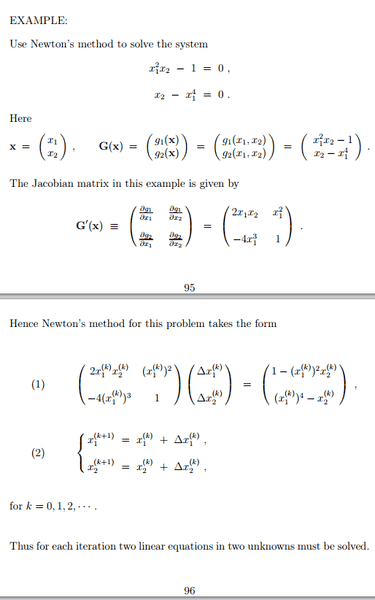# Program to solve system of nonlinear equations

twoski

## Homework StatementSolve the aforementioned system of nonlinear equations using newton's method. write a program to carry out the calculations (it must use gauss elimination).

Use the values 0-3 for $x_{1}^{(0)}, x_{2}^{(0)}$ (ie. 16 data sets total).

## The Attempt at a Solution

So i have hit my first roadblock: For $x_{1}^{(0)}=0, x_{2}^{(0)}=0$ this makes absolutely no sense.

You end up with $0 * Δx_{1}^{(0)} + 0 * Δx_{2}^{(0)} = 1$ which is impossible. What the heck?

Homework Helper
Gold Member
You will always run into problems with Newton's method if one of the iteration points is stationary, which happens whenever the Jacobian matrix is singular (##\det \mathbf{G}'(\mathbf{x}) =0##). In this case there is a line of stationary points on the ##x_2## axis, so several of the starting points listed will fail. There are alternative methods to Newton, but they would seem to be beyond the scope of the problem.

twoski
Correct, i am supposed to use newton's method with gauss elimination specifically... So should i email my prof about this? Is there some sort of method to solving this case?

Homework Helper
Gold Member
Correct, i am supposed to use newton's method with gauss elimination specifically... So should i email my prof about this? Is there some sort of method to solving this case?

Sure, you could email the prof or grader. If your course or text didn't cover the methods for singular matrices, I don't think that you'd be expected to learn them just to do this problem. If you are interested in reading about one method, you might check out this chapter that uses Singular Value Decomposition (SVD) of the Jacobian to improve the Newton method. There's a similar (maybe equivalent) method described in this paper, but the presentation is more technical than the 1st reference. Again, I am certain you are not expected to use these methods, but it can't hurt to check with the prof.

twoski
I messaged the prof and he tells me,

Just report your findings (with a brief discussion). I am assuming that you get convergence for at least some of the initial data. You can take some liberty with the initial data ...

Not sure what "some liberty" means but i did manage to make my program work for cases where the matrix doesn't have a row of zeros.

Homework Helper
Gold Member
Instead of starting with a bad point on the list, you could choose an initial point a small distance away from the suggested one to see if the convergence improves.

twoski
Ah, so in place of 0, i could take, say, 0.001 and try with that?